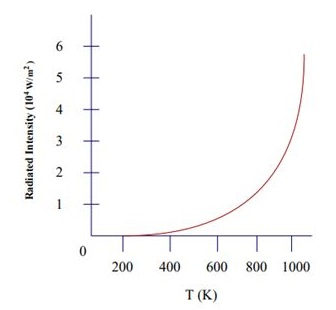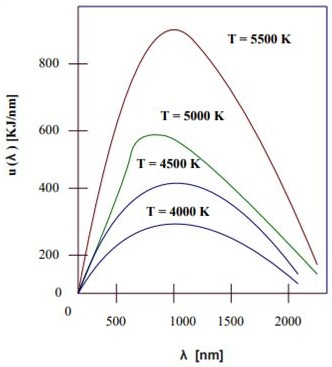# Stefan Boltzmann Constant

## Introduction

The constant of Stefan Boltzmann is used to calculate the heat amount that is radiated by the blackbody. It preoccupied all the radiant energy that strikes it and releases the total radiated energy. In understanding the constant of Stefan Boltzmann's law, the blackbody is crucially important because that discusses the amount of power the sun provides off the provided temperature.

This law constantly helps in analysing and examining the amount of heat and energy released by the earth into space. It permits scientists to examine and identify the amount of hotness of the sun that is based on the striking power of the Earth in a square metre. Boltzmann constantly emerges from the name of Austrian Physicist Ludwig Boltzmann.

## What is Stefan Boltzmann’s Law?

Stefan Boltzmann’s law states that the ultimate radiant power of heat released from the surface is comparable to the fourth power of the complete temperature. The law applies to black bodies and theoretical surfaces that engrossed all the incidents of radiation or heat (Montambaux, 2018). The law of Stefan Boltzmann was formulated in the year 1879 by Josef Stefan, an Austrian physicist as an outcome of experiential studies.

Images Coming soon

The ultimate energy released per unit area of blackbody surfaces across different per unit time wavelengths is directly comparable to the fourth power of the thermodynamic temperature of the blackbody. It is considered by physicists that E is represented as the radiant energy of heat released from a unit area per second and T is presented as the absolute temperature in Kelvin (Santos & Khanna, 2019). It is obtained by the experimental study that $\mathrm{E=\sigma T^{4}}$, where $\mathrm{\sigma}$ is referred to as the proportionality constant.

Blackbody radiation is defined as the radiation created by heated materials, especially from the blackbody. A blackbody is referred to as object or material that sucks up all the radiation such as visible light, ultraviolet light and infrared light. Blackbodies are generally utilised for heating, security, lighting, testing, thermal imaging and measurement applications (Lebelo et al. 2018). A blackbody is dark at room temperature; nevertheless, it brightens at the reflected wavelength at the maximum temperature.Everything in a blackbody brightens relies on the temperature. Hotter means more bright light in the context of blackbody radiation. In this radiation, the interaction of colour of the brightening features of an object and temperature is easily comprehended.

Consider an instance of heated metal which genuinely changes light colour. As opined by Naboni et al. (2019), a colour transformation of metals releases from red to orange to yellow as it reaches high. It is known that blackbody radiation is released as the object is hot because of more collisions of atoms and particles. More violent collisions mean more visibility of radiation. This is the main reason things get brighter with more heat.

## What is Absolute Temperature?

Absolute temperature is referred to as the temperature that is calculated utilising the scale of Kelvin where zero is mentioned as absolute zero. The point of zero is demonstrated as the temperature at which the matter particles have minimal motion and can get no colder (Bityukov, Khudak & Gusein-Zade, 2018). Absolute temperature is also known as thermodynamic temperature.There is a difference between temperature and absolute temperature. Absolute temperature represents the extremely low temperature at which the system is in its probable lowest state of energy (Henry & Merlis, 2019). On the other hand, heat intensity present in an object or a substance is termed temperature. The Fahrenheit scale or Rankine scale has the highest degree interval which is another scale of absolute temperature.

## The Formula for Stefan Boltzmann constant

The Stefan Boltzmann constant value is determined and derived experimentally which is derived from the Boltzmann constant.

$$\mathrm{\sigma =\frac{2\pi ^{5}K_{B}^{4}}{15h^{3}c^{2}}=5.670367(13)\times 10^{-8}J.m^{2}.S^{-1}.K^{-4}}$$

According to this formula, $\mathrm{K_{B}}$ is the Boltzmann constant and h is represented as the Planck constant. C is represented as light speed and $\mathrm{\sigma}$ is the proportionality constant.

## Application of Stefan Boltzmann law

In the physical world, the constant of Stefan Boltzmann is wide which are

• It is used to calculate the heat amount radiated from the dark body of any object.

• It can be utilised for converting the temperature to the units for the intensity which is ideally power per unit area.

## Conclusion

Stefan's law and its constant are very famous in astrophysics but it is regarded as an essential connection. It can be derived from Planck's law and thermodynamics law. A similar law like Stefan's law has been derived in the year 1884 by Ludwig Boltzmann from the considerations of thermodynamics. The blackbody is a perfect absorber which is the main element of Stefan Boltzmann's constant. This constant of Stefan Boltzmann is similar to that of the gas ratio constant to the Avogadro constant.

## FAQs

Q.1. What is the unit type for Stefan Boltzmann constant value?

Ans. The constant of Stefan Boltzmann is accepted and provided in SI units.

Unit type Value for Stefan Boltzman’s constant Units
CGS units $\mathrm{\sigma \approx 5.6704\times 10^{5}}$ $\mathrm{erg.cm^{2}.s^{1}.K^{4}}$
US customary units $\mathrm{\sigma \approx 1.714\times 10^{9}}$ $\mathrm{BTU.hr^{1}.ft^{2}.^{\circ}R^{4}}$
Thermochemistry $\mathrm{\sigma \approx 11.7\times 10^{8}}$ $\mathrm{cal.cm^{2}.day^{1}.K^{4}}$

Q.2. What denotes the SI unit of Stefan Boltzmann constant?

Ans. The SI unit of constant of Stefan Boltzmann is represented as $\mathrm{W.m^{-2}.K^{-4}}$. In CGS unit it is expressed as an erg. $\mathrm{cm^{2}}$.

Q.3. Define the utilisation of Stefan’s law?

Ans. The law of Stefan Boltzmann illustrates the amount of power the Sun is given as the temperature. The principle of radiation of energy per second by area of the unit of a blackbody at the temperature (T) of thermodynamics is directly comparable to $\mathrm{T^{4}}$.

Q.4. What is defined as the Stefan equation?

Ans. In the equation, Stefan discusses the reliance of the thickness of ice cover on the history of temperature. Particularly, ice accretion is directly comparable to the number's square root of the degree days below freezing.